Courses

# Baye’s Theorem - Probability, Business Mathematics and Statistics B Com Notes | EduRev

## B Com : Baye’s Theorem - Probability, Business Mathematics and Statistics B Com Notes | EduRev

The document Baye’s Theorem - Probability, Business Mathematics and Statistics B Com Notes | EduRev is a part of the B Com Course Business Mathematics and Statistics.
All you need of B Com at this link: B Com

This theorem is associated with the name of Reverend Thomas Bayes. It is also known as the inverse probability. Probabilities can be revised when new information pertaining to a random experiment is obtained. One of the important applications of the conditional probability is in the computation of unknown probabilities, on.the basis of the information supplied by the experiment or past records. That is, the applications of the results of probability theory involves estimating unknown probabilities and making decisions on the basis of new sample information. This concept is referred to as Bayes’ Theorem. Quite often the businessman has the extra information on a particular event, either through a personal belief or from the past history of the events. Revision of probability arises from a need to make better use of experimental information. Probabilities assigned on the basis of personal experience, before observing the outcomes of the experiment are called prior probabilities. For example, probabilities assigned to past sales records, to past number of defectives produced by a machine, are examples of prior probabilities. When the probabilities are revised with the use of Bayes’ rule, they are called posterior probabilities. Bayes’ theorem is useful in solving practical business problems in the light of additional information. Thus popularity of the theorem has been mainly because of its usefulness in revising a set of old probability (Prior Probability) in the light of additional information made available and to derive a set of new probabilily (i.e. Posterior Probability)

Bayes’ Theorem : An event  A can occurre only if one of the mutually exclusive and exhaustive set of events B1, B2,  ..... Bn occurs. Suppse that the unconditional probabilities

P(B1), P(B2), .... P(Bn)

and the conditional probabilities

P(A/B1), P(A/B2), .... P(A/Bn)

are known. Then the conditional probability P(Bi/A) of a specific event Bi, when A is stated to have actually accquared, is given by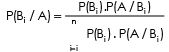This is known as Bayes’ Theorem.

The following  example illustrate the  application of Baye’s Theorem.

The above calculation can be verified as follows :

If 1,000 scooters were produced by the two plants in a particular week, the number of scooters produced by Plant I & Plant II are respectively :

1,000 x 80% = 800 scooters
1,000 x 20% = 200 scooters

The number of standard quality scooters produced by Plant I : 800 x 85/100 = 680 scooters

The number of standard quality scooters produced by Plant II : 200 x 65/100 = 130 Scooters.

The probability that a standard quality scooter was produced by Plant I is :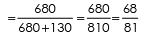The probability that a standard quality scooter was produced by Plant II is :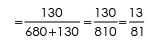The same process i.e. revision can be repeated if more information is made available. Thus it is a good theorem in improving the quality of probability in decision making under uncertainty.

Example 16 :

You note that your officer is happy on 60% of your calls, so you assign a probability of his being happy on your visit as 0.6 or 6/10. You have noticed also that if he is happy, he accedes to your request with a probability of 0.4 or 4/10 whereas if he is not happy, he acedes to the request with a probability of 0.1 or D
or 1/10 You call one day, and he accedes to your request. What is the probability of his being happy ?
Solution
:

Let- H be the Hypothesis that the officer is happy and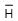the Hypothesis that the officer is not happy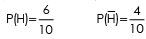Let A be the event that he accedes to request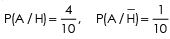To find P(H/A), according to Baye’s Theorem,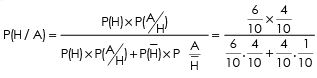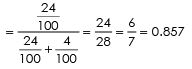Example 17 :

A company has two plants to manufacture scooters. Plant I manufactures 80% of the scooters and plant II manufactures 20%. At Plant I, 85 out of 100 scooters are rated standard quality or better. At Plant II, only 65 out of 100 scooters are rated standard quality or better. What is the probability that the scooter selected at random came from Plant I if it is known that the scooter is of standard quality ?
What is the probability that the scooter came from Plant II if it is known that the scooter is of standard quality.

Solution :

Let A1 be the event of drawing a scooter produced by Plant I and A2 be the event of drawing a scooter produced by Plant II. B be the event of drawing a standard quality scooter produced by either Plant I or Plant II Then, from the first information :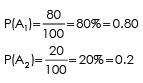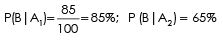The required values are computed in the following table :

 Event Prior Probabifity(2) Condrrionaf Probability(3) Join t Probability (4) Posterior Probability (Revised)(5) [4 ÷ P(B}] A1 0.80 0.85 0.68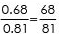A2 0.20 0.65 0.13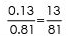1 P(B) = 0.81 1

From the first information we may say that the standard scooter is drawn   from   Plant   I   since   P(A1) = 80% which is greater than P(A2) = 20%,

From the additional information i.e. at Plant I, 85 out of 100 and at Plant II 65 out of 100 are rated standard quality, we can give better answer, Thus we may conclude that the standard quality of scooter is more likely drawn from the output by Plant I.

Offer running on EduRev: Apply code STAYHOME200 to get INR 200 off on our premium plan EduRev Infinity!

122 videos|142 docs

,

,

,

,

,

,

,

,

,

,

,

,

,

,

,

,

,

,

,

,

,

,

,

,

;